Wir verwenden Google für unsere Suche. Mit Klick auf „Suche aktivieren“ aktivieren Sie das Suchfeld und akzeptieren die Nutzungsbedingungen.

# New in SG-Lib 4.0

###### SGTdrill(SG,N,t,CPL)- drills a contour hole into a solid geometries frame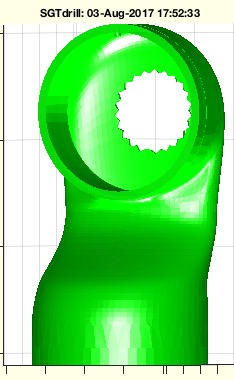% SGTdrill(SG,N,t,CPL) - drills a contour hole into a solid geometries frame % (by Tim Lueth, VLFL-Lib, 2017-AUG-03 as class: SURFACES)% % Introduced first in SolidGeometry 4.0% % See also: SGbool4, SGT% % SG=SGTdrill(SG,[N,t,CPL])% === INPUT PARAMETERS ===% SG: Solid Geometry% N: Name of Frame; default is 'B'% t: depth of hole% CPL: radius or contour % === OUTPUT RESULTS ======% SG: Solid Geometry with hole% % EXAMPLE: % A=SGbox([30,20,10]); A=SGTset(A,'B',TofP([0 0 5]))% SGTdrill(A,'',1,5)% % % See also: SGbool4, SGT% % % Copyright 2017 Tim C. Lueth
###### copyfig (cf)- copies a axis diagramm into a new MATLAB figure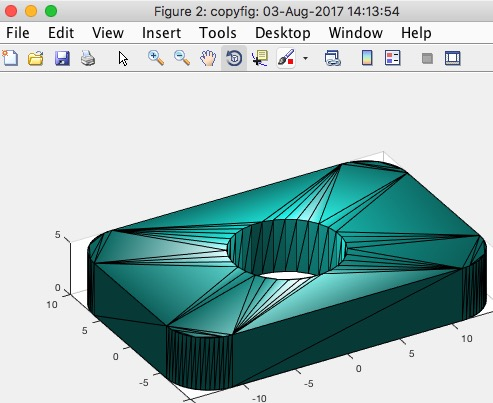% copyfig (cf) - copies a axis diagramm into a new MATLAB figure% (by Tim Lueth, VLFL-Lib, 2017-AUG-03 as class: USER INTERFACE)% % Menue, colors, grid, etc. are not copied. (Status of: 2018-08-15)% % Introduced first in SolidGeometry 4.0% % See also: copyplot, snapplot, copyobj, movegui, subfig% % copyfig([cf])% === INPUT PARAMETERS ===% cf: copy figure handle; default is gcf/gca% % EXAMPLE: % SGfigure(SGsample(27)); view(-30,30);% copyfig% % See also: copyplot, snapplot, copyobj, movegui, subfig% % % Copyright 2017-2018 Tim C. Lueth
###### SGcutT(SG,T,centxy)- cuts an object below a arbitrary plane using SGcut relative to the center of floor of bb% SGcutT(SG,T,centxy) - cuts an object below a arbitrary plane using SGcut relative to the center of floor of bb% (by Tim Lueth, VLFL-Lib, 2017-AUG-02 as class: SURFACES)% % Beside Franz Irlinger who introduce me into the beauty of mechanism % instead of robots, I'd like to thank Marcus Rompf and Henning Schober, % both involved into the design of accordion mechanics. (Status of: % 2018-08-21)% % Introduced first in SolidGeometry 4.0% % See also: SGcut, SGcut2, SGcutBB% % [SGU,SGO]=SGcutT(SG,T,[centxy])% === INPUT PARAMETERS ===% SG: Solid Geoemtry% T: cutting plane to cut under% centxy: if true, center the SG on xy-plane; default=false | new in 4.1% === OUTPUT RESULTS ======% SGU: New solid geometry% SGO: % % EXAMPLE: % load JACO_robot.mat% SGcutT(JC0,TofR(rot(pi/6,pi/6,pi/6),[0 0 100]),true)% SGcutT(JC0,TofR(rot(pi/6,pi/6,pi/6),[0 0 100]))% % See also: SGcut, SGcut2, SGcutBB% % % Copyright 2017-2018 Tim C. Lueth
###### cputoc- similar to toc but uses the cpu timer% cputoc - similar to toc but uses the cpu timer% (by Tim Lueth, VLFL-Lib, 2017-AUG-01 as class: AUXILIARY PROCEDURES)% % Introduced first in SolidGeometry 4.0% % See also: cputic, cputime, timeit% % tc=cputoc% === OUTPUT RESULTS ======% tc: Elapsed cpu time in seconds% % EXAMPLE: % tic; for i=10000000000; x=cos(pi/16); end; toc% cputic; for i=10000000000; x=cos(pi/16); end; cputoc% f=@() cos(pi/3), timeit(f)% % See also: cputic, cputime, timeit% % % Copyright 2017 Tim C. Lueth
###### cputic- similar to tic but uses the cpu timer% cputic - similar to tic but uses the cpu timer% (by Tim Lueth, VLFL-Lib, 2017-AUG-01 as class: AUXILIARY PROCEDURES)% % Introduced first in SolidGeometry 4.0% % See also: cputoc, cputime, timeit% % cputic% % EXAMPLE: % tic; for i=10000000000; x=cos(pi/16); end; toc% cputic; for i=10000000000; x=cos(pi/16); end; cputoc% f=@() cos(pi/3), timeit(f)% % See also: cputoc, cputime, timeit% % % Copyright 2017 Tim C. Lueth
###### collofBBs(bba,bbb)- returns from two bounding box list the colliding index lists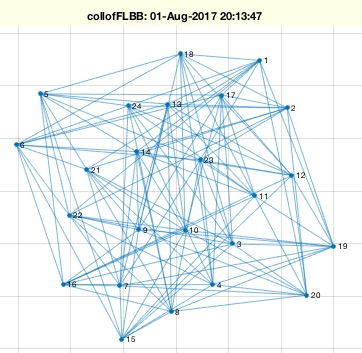% collofBBs(bba,bbb) - returns from two bounding box list the colliding index lists% (by Tim Lueth, VLFL-Lib, 2017-AUG-01 as class: ANALYZING PROCEDURES)% % This fnctn can be used directly to preprocess, i.e. accelerate facet % crossing fnctns (Status of: 2017-08-01)% % Introduced first in SolidGeometry 4.0% % See also: VLDLBBofVLFL% % [CL,ca,cb]=collofBBs(bba,bbb)% === INPUT PARAMETERS ===% bba: bounding box list A% bbb: bounding box list B% === OUTPUT RESULTS ======% CL: Crossing list [n x 2] = [ia ib]% ca: optional list of crossing boxes in A; ca=unique(CL(:,1),'stable'); % cb: optional list of crossing boxes in B; cb=unique(CL(:,2),'stable');% % EXAMPLE: % A=SGbox([30,20,10]);% [~,~,~,~,~,~,bb]=VLDLBBofVLFL(A.VL,A.FL,1)% collofBBs(bb,bb)% % See also: VLDLBBofVLFL% % % Copyright 2017 Tim C. Lueth
###### SGbool4(flag,A,B,thr)- returns the result of a boolean operation on two closed elementary solids% SGbool4(flag,A,B,thr) - returns the result of a boolean operation on two closed elementary solids% (by Tim Lueth, VLFL-Lib, 2017-AUG-01 as class: SURFACES)% % ATTENTION: SGbool4 is the same fnctn as SGbool3, but much faster. It % uses the - now public - fnctns % SGintersectFacetPoints and VLFLinsertFacetPoints which were private in % SGbool3. It has a more clear structure, but has exactly the same % algorith for face assignment. In addition it shows all four possible % results of SGbool for two solids. Use CSGsample for testing. % Nevertheless, it should be clear that there are limitations even of % closed surface models with SGbool3 and SGBool4: % 1. Never have identical points in both surfaces% 2. Never have identical lines in both surfaces% 3. Never have indentical surface planes in both surfaces% 4. Never have an overlap of enclosed "holes" => unmotivated surfaces % If there is a risc, use SGgrow to grow before substraction or addition % by 1e-4% It is possible to remove those problems one day by cutting tetrahedrons % instead of triangles% A => A without B% B => B without A% + => A combined with B% x => A intersected with B% (Status of: 2017-08-08)% % Introduced first in SolidGeometry 4.0% % See also: SGbool, SGbool2, SGbool3% % SGX=SGbool4(flag,A,B,[thr])% === INPUT PARAMETERS ===% flag: Boolean operator ('AB+x')% A: Solid A (VL/FL)% B: Solid B (VL/FL)% thr: threshold for grid; default is 1e-5% === OUTPUT RESULTS ======% SGX: resulting surface geometry% % EXAMPLE: % [A,B]=CSGsample(1); SGbool4('-',A,B);% [A,B]=CSGsample(2); SGbool4('-',A,B);% [A,B]=CSGsample(3); SGbool4('-',A,B); % Problems with crossing holes% [A,B]=CSGsample(4); SGbool4('-',A,B);% % % See also: SGbool, SGbool2, SGbool3% % % Copyright 2017 Tim C. Lueth
###### VLDLBBofVLFL(VL,FL,thr)- returns for a facet list the vertex coordinates, the edge vectors, and the bounding box of the facets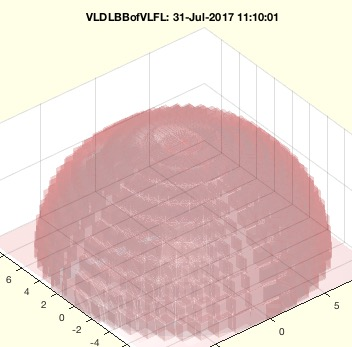% VLDLBBofVLFL(VL,FL,thr) - returns for a facet list the vertex coordinates, the edge vectors, and the bounding box of the facets % (by Tim Lueth, VLFL-Lib, 2017-JUL-31 as class: SURFACES)% % This fnctn returns for a facet list the vertex coordinates, the edge % vectors, and the bounding box of the facets. Is also used in some other % fnctn with global variables.% The bounding box list can be used for fast comparison of facets before % a crossing check (Status of: 2017-08-01)% % Introduced first in SolidGeometry 4.0% % See also: crossingfacets2VLFL, collofBBs% % [v1,v2,v3,d1,d2,d3,bb]=VLDLBBofVLFL(VL,FL,[thr])% === INPUT PARAMETERS ===% VL: Vertex list% FL: Facet List% thr: supplement to bounding boxes; default is 1e-12% === OUTPUT RESULTS ======% v1: List of first vertex of facet list: v1=VL(FL(:,1),:);% v2: List of second vertex of facet list: v2=VL(FL(:,2),:);% v3: List of thried vertex of facet list: v3=VL(FL(:,3),:);% d1: d1=v2-v1;% d2: d2=v3-v2;% d3: d3=v1-v3;% bb: bounding box of all facets;% % EXAMPLE: % A=SGsample(18); VLDLBBofVLFL(A.VL,A.FL);% A=SGsample(19); VLDLBBofVLFL(A.VL,A.FL);% A=SGsample(27); VLDLBBofVLFL(A.VL,A.FL);% % See also: crossingfacets2VLFL, collofBBs% % % Copyright 2017 Tim C. Lueth
###### CPLdisttopoint(CPL,p);- returns the minmal distance between a contour and a given point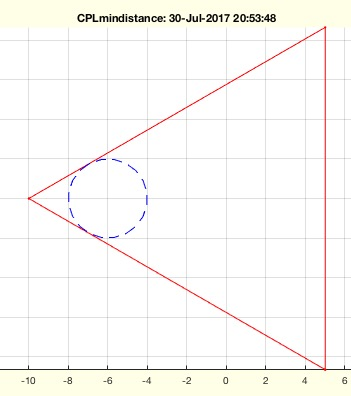% CPLdisttopoint(CPL,p); - returns the minmal distance between a contour and a given point% (by Tim Lueth, VLFL-Lib, 2017-JUL-30 as class: ANALYTICAL GEOMETRY)% % for each edge/ conenction between two points, the distance to the % origin or the optional point p is calculated (Status of: 2017-07-30)% % Introduced first in SolidGeometry 4.0% % See also: centerCPL% % LITERATURE:% https://en.wikipedia.org/wiki/Distance_from_a_point_to_a_line% % dmin=CPLdisttopoint(CPL,[p]);% === INPUT PARAMETERS ===% CPL: Closed polygon line% p: point of interest; default is [0 0]% === OUTPUT RESULTS ======% dmin: radius of the minmal circle that touches the contour% % EXAMPLE: % CPLdisttopoint(CPLofPL(PLcircle(10,3)),[-6 0])% % See also: centerCPL% % % Copyright 2017 Tim C. Lueth
###### SGtorquelimiter(R,m,t,h)- returns a torque limiter part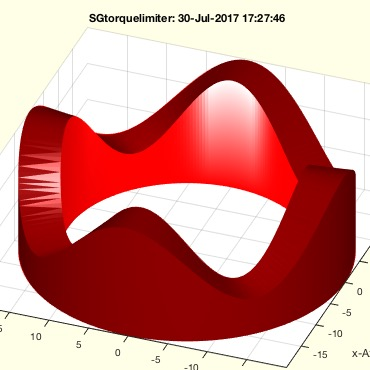% SGtorquelimiter(R,m,t,h) - returns a torque limiter part% (by Tim Lueth, VLFL-Lib, 2017-JUL-30 as class: MODELING PROCEDURES)% % Introduced first in SolidGeometry 4.0% % See also: VLFLsnaprivet% % SG=SGtorquelimiter([R,m,t,h])% === INPUT PARAMETERS ===% R: Radius [Router Rinner]% m: modul; number of fnctns / cosinus% t: amplitude of cosinus% h: height of the solid% === OUTPUT RESULTS ======% SG: Solid geometry% % See also: VLFLsnaprivet% % % Copyright 2017 Tim C. Lueth
###### zigzag(x,per)- returns a zig zag curve in the interval [0 2*pi]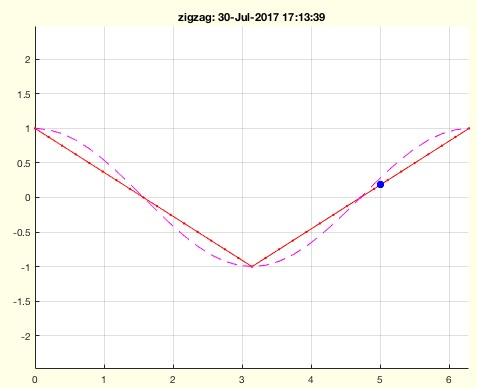% zigzag(x,per) - returns a zig zag curve in the interval [0 2*pi]% (by Tim Lueth, VLFL-Lib, 2017-JUL-30 as class: ANALYTICAL GEOMETRY)% % corresponds to cosinus fnctn (Status of: 2017-07-30)% % Introduced first in SolidGeometry 4.0% % See also: sin, cos% % y=zigzag(x,[per])% === INPUT PARAMETERS ===% x: x value% per: periode; default is 2*pi% === OUTPUT RESULTS ======% y: zigzag value% % EXAMPLE: % zigzag(0)% zigzag(0:2*pi)% zigzag(1,4)% % See also: sin, cos% % % Copyright 2017 Tim C. Lueth
###### SGTrotate(SG,N,ax,val)- returns a solid rotated around a Frame coordinate System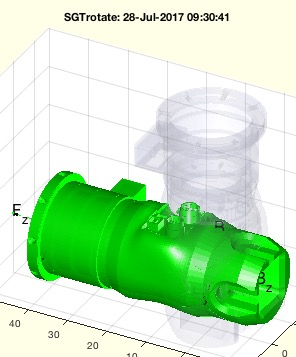% SGTrotate(SG,N,ax,val) - returns a solid rotated around a Frame coordinate System% (by Tim Lueth, VLFL-Lib, 2017-JUL-28 as class: KINEMATICS AND FRAMES)% % Introduced first in SolidGeometry 4.0% % See also: SGtransT, SGrotate% % SGN=SGTrotate(SG,[N,ax,val])% === INPUT PARAMETERS ===% SG: Solid Geoemtry% N: Frame; default is ez; nan means eigenvectors; default is 'B'% ax: rotation axis sequence; 'xyz' or 'x' or 'z'% val: values for rotation; same as ax% === OUTPUT RESULTS ======% SGN: Spatial rotated Solid includeing the frames% % See also: SGtransT, SGrotate% % % Copyright 2017 Tim C. Lueth
###### SGplugofSGT(SG,N,h,ms,tol)- creates a plug for an SGT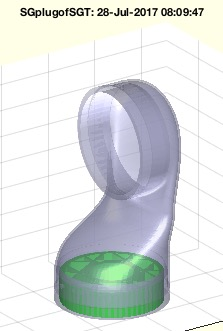% SGplugofSGT(SG,N,h,ms,tol) - creates a plug for an SGT% (by Tim Lueth, VLFL-Lib, 2017-JUL-28 as class: SURFACES)% % creates a plug for feature surface by orthogonal walls and leaves space % for a magnetic-connection. The plug is a honeycomp structure with a % rigid border that fits into the opening of the frame related surface. % In the center (Status of: 2017-07-29)% % Introduced first in SolidGeometry 4.0% % See also: JACOmodel% % SGP=SGplugofSGT(SG,N,[h,ms,tol])% === INPUT PARAMETERS ===% SG: Solid geoemtry with frames% N: Name of frame, the plug is for% h: Optional height of plug; default is 5mm% ms: Diameter of a magnet; default is 10mm% tol: Tolerance for all values; default is 0.2mm (smaller)% === OUTPUT RESULTS ======% SGP: Solid Geometry of the Plug% % See also: JACOmodel% % % Copyright 2017 Tim C. Lueth
###### exp_2017_07_27 (SG)- EXPERIMENT to compare the pdegplot(model,'Facelabel','on') with FSplot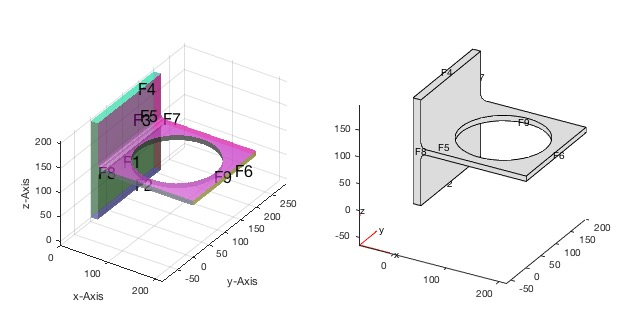% exp_2017_07_27 (SG) - EXPERIMENT to compare the pdegplot(model,'Facelabel','on') with FSplot% (by Tim Lueth, VLFL-Lib, 2017-JUL-27 as class: EXPERIMENTS)% % currently it looks identically. May be same strategy and the same % alhpha angle of ~1 (60 degree) (Status of: 2017-07-27)% % Introduced first in SolidGeometry 4.0% % exp_2017_07_27([SG])% === INPUT PARAMETERS ===% SG: Solid Geometry% % EXAMPLE: % SG=SGreadSTL(which('BracketWithHole.stl'),1000);% % % % % % Copyright 2017 Tim C. Lueth
###### FSplot(TR,alp,fsi,col,siz);- plots the featureEdges of TR, SG or VLFL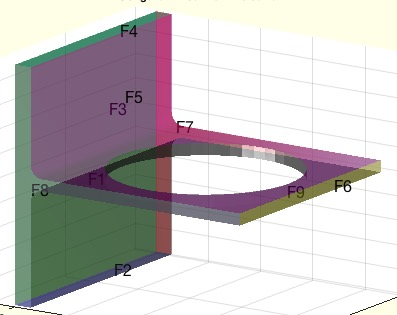% FSplot(TR,alp,fsi,col,siz); - plots the featureEdges of TR, SG or VLFL% (by Tim Lueth, VLFL-Lib, 2017-JUL-27 as class: VISUALIZATION)% % Similar to FE plot, it plots the feature surfaces of a solid;% Feature surfaces are not officially supported by matlab, nevertheless % there the pde toolbox for instance has a facet plot, which is % comparable to FS.% In contrast to pdeplotfaces, the SG-Lib gives you full access to the % feature surfaces using surfacesofSG. Especially for automatic design it % is necessary to select surfaces in many different ways, such as a % normal vector. Also information on the faces are required. (Status of: % 2017-08-25)% % Introduced first in SolidGeometry 4.0% % See also: FEplot, surfacesofSG, textVLFS, pdeplotfaces, pdegplot% % h=FSplot([TR,alp,fsi,col,siz]);% === INPUT PARAMETERS ===% TR: surface (Tetrahedron, Triangulation, Solid)% alp: alpha value for neigbor facets% fsi: selected FS; depend on alpha; default is all% col: color of FS; default is colofn% siz: size of descriptiors; default is 16% === OUTPUT RESULTS ======% h: handle to graphics object consisting of text and patches% % EXAMPLE: FSplot(SGsample(3))% SG=SGreadSTL(which('BracketWithHole.stl'),1000);% SGfigure; FSplot(SG,1)% SGfigure; FSplot(SG,0.1);% SGfigure; FSplot(SG,1,[4 6]);% SGfigure; FSplot(SG,1,[4 6],'r');% SGfigure; FSplot(SG,1,[4 6],'r',30);% % See also: FEplot, surfacesofSG, textVLFS, pdeplotfaces, pdegplot% % % Copyright 2017-2018 Tim C. Lueth
###### csgofPL(PL,sf)- returns MATLAB's Constructed Solid Geometry (CSG) dg matrix for a list of polygons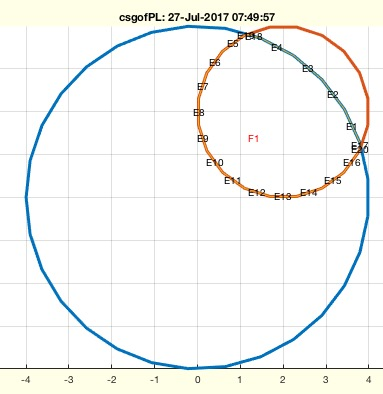% csgofPL(PL,sf) - returns MATLAB's Constructed Solid Geometry (CSG) dg matrix for a list of polygons% (by Tim Lueth, VLFL-Lib, 2017-JUL-26 as class: CLOSED POLYGON LISTS)% % TIP: Currently I do not see an advantage to use csg Constructed Solid % Geometry (CSG) instead of boundary Representation (BREP) which is the % foundation of the SG-Lib. Nevertheless, an interface should exist.% % Matlab supports for 2D geometries the method Constructed Solid % Geometries (CSG) Decompositon already before 2006a. This fnctn converts % a Point List into a csg or gd (Geometric Description Matrix).% It is possible either to convert a PL into the 2D csg format for one % polygon or% It is possible to convert a cell list of Polygon plus a string formular % into a geoemetric matrix.% Currently the first polygon is "A", the second "B" and so on.% The Formula is a string of Type "A+B" or "A-B" "A*B" or "A" or "B". % (Status of: 2017-07-27)% % Introduced first in SolidGeometry 4.0% % See also: decsg, pdemodel, geometryFromEdges, pdegeom, CPLpolybool% % [csg,bt]=csgofPL(PL,[sf])% === INPUT PARAMETERS ===% PL: CPL of cell list of CPLs% sf: Formula wrt. to A polygon sequence 'A' 'B' 'C' etc.% === OUTPUT RESULTS ======% csg: or dg (Geometry description matrix)% bt: % % EXAMPLE: % csgofPL(PLstar(10)); % returns matlab's CSG polygon list format% csgofPL({PLstar(10)},'A') % returns the geometry list of 1st Polygon% csgofPL({PLcircle(4);PLcircle(2)+2},'A')% csgofPL({PLcircle(4);PLcircle(2)+2},'B')% csgofPL({PLcircle(4);PLcircle(2)+2},'A+B')% csgofPL({PLcircle(4);PLcircle(2)+2},'A-B')% % % See also: decsg, pdemodel, geometryFromEdges, pdegeom, CPLpolybool% % % Copyright 2017 Tim C. Lueth
###### CPLradialEdges(CPL,R)- returns a vertex list (VL) with rounded edges% CPLradialEdges(CPL,R) - returns a vertex list (VL) with rounded edges % (by Tim Lueth, VLFL-Lib, 2017-JUL-26 as class: ANALYZING PROCEDURES)% % ATTENTION: This fnctn is based on VLradialEdges not on PLradialEdges% Replaces edges by a radial curve. The desired radius can be reduced % automatically if necessary. It is possible to maximize the radius % automatically.% In case that R is negative; VLbreakEdges is used instead (Status of: % 2017-07-26)% % Introduced first in SolidGeometry 4.0% % See also: PLradialEdges, SGcontourtube, RLofEulerInterpolation, % VLinsertEulerSteps, VLradialEdges, TofPez, PLtangentcirc, % VLtangentcirc, SGbreakCorners, SGradialCorners% % CPLN=CPLradialEdges(CPL,[R])% === INPUT PARAMETERS ===% CPL: Vertex list nx3% R: Radius; default is none% === OUTPUT RESULTS ======% CPLN: New closed Polygon list; All points are doubled% % EXAMPLE: % CPLradialEdges(CPLsample(4),5);% CPLradialEdges(CPLsample(12),5);% CPLradialEdges(CPLsample(12),-4);% % See also: PLradialEdges, SGcontourtube, RLofEulerInterpolation, % VLinsertEulerSteps, VLradialEdges, TofPez, PLtangentcirc, % VLtangentcirc, SGbreakCorners, SGradialCorners% % % Copyright 2017 Tim C. Lueth
###### SGchamfer_org(SG,ph,ed,r,stype)- chamfers the edges of a 2.5D Solid Geometry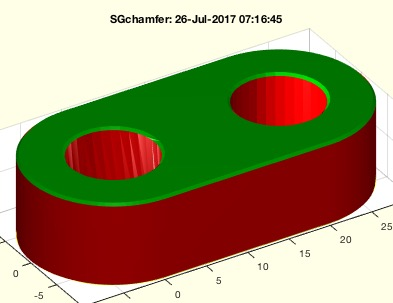% SGchamfer_org(SG,ph,ed,r,stype) - chamfers the edges of a 2.5D Solid Geometry% (by Tim Lueth, VLFL-Lib, 2017-JUL-26 as class: SURFACES)% % It does not returns as perfect edges as SGofCPLzchamfer (Status of: % 2017-08-16)% % Introduced first in SolidGeometry 4.0% % See also: SGofCPLzchamfer, SGof2CPLzheurist, CPLradialEdges% % % SGN=SGchamfer_org(SG,[ph,ed,r,stype])% === INPUT PARAMETERS ===% SG: Solid Geometry with just two different z values% ph: edge/phase size default is 0.3% ed: curved edges; default is true% r: radius for radial edges; default is 0% stype: default is 'length'; see SGof2CPLzheurist% === OUTPUT RESULTS ======% SGN: % % EXAMPLE: % SGchamfer(SGsample(2))% SGchamfer(SGofCPLz(PLcircleoval(10,'',20,5),10))% SGchamfer(SGofCPLz(PLstar(10),10),0.3,'')% SGchamfer(SGofCPLz(PLstar(10),10),0.3,'',2)% SGchamfer(SGofCPLz(PLstar(10),10),0.3,'',-0.5)% % See also: SGofCPLzchamfer, SGof2CPLzheurist, CPLradialEdges% % % % Copyright 2017-2018 Tim C. Lueth
###### CPLfillin(CPL,Amin,Asam)- creates a contour that fills a another or several contours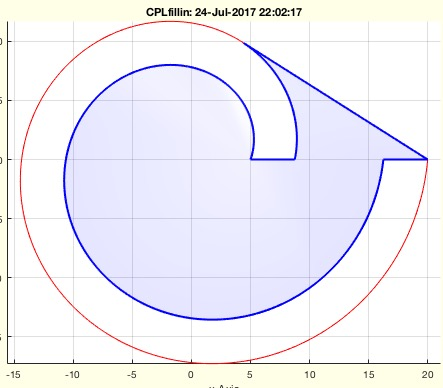% CPLfillin(CPL,Amin,Asam) - creates a contour that fills a another or several contours % (by Tim Lueth, VLFL-Lib, 2017-JUL-24 as class: CLOSED POLYGON LISTS)% % Powerful fnctn that is not the same as CPLpolybool! (Status of: % 2017-07-25)% % Introduced first in SolidGeometry 4.0% % See also: delaunayofCPL% % [iCPL,A]=CPLfillin(CPL,[Amin,Asam])% === INPUT PARAMETERS ===% CPL: Closed Polygon List% Amin: Minimum surface to be interested% Asam: percentage of maximum area ; default a=90%, 0.9% === OUTPUT RESULTS ======% iCPL: inverse polygon% A: VL and FL of the open surface% % EXAMPLE: % CPLfillin(CPLsample(14));% CPLfillin(CPLsample(19));% CPLfillin(CPLsample(21));% % See also: delaunayofCPL% % % Copyright 2017 Tim C. Lueth
###### findchangerow(c)- returns in a list of succeding rows, when the row is different to its predecessor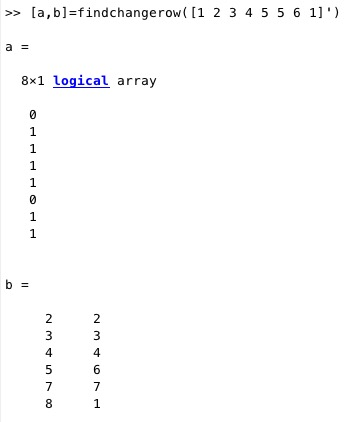% findchangerow(c) - returns in a list of succeding rows, when the row is different to its predecessor% (by Tim Lueth, VLFL-Lib, 2017-JUL-24 as class: AUXILIARY PROCEDURES)% % creates a change index list from any row list (Status of: 2017-07-24)% % Introduced first in SolidGeometry 4.0% % See also: mod1% % [i,CIL]=findchangerow(c)% === INPUT PARAMETERS ===% c: list of succeeding rows% === OUTPUT RESULTS ======% i: index list% CIL: Change index list% % EXAMPLE: % [a,b]=findchangerow([1 2 3 4 5 5 6 1]')% % See also: mod1% % % Copyright 2017 Tim C. Lueth
###### CPLofSGimageslice(SG,z,l,t)- returns a slice produced by an orthographic matlab figure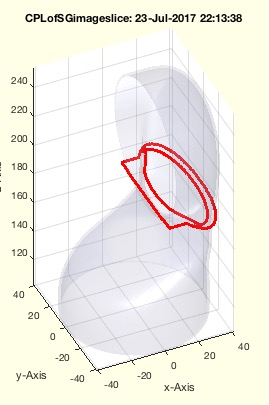% CPLofSGimageslice(SG,z,l,t) - returns a slice produced by an orthographic matlab figure% (by Tim Lueth, VLFL-Lib, 2017-JUL-23 as class: CLOSED POLYGON LISTS)% % This fnctn is neither faster nor more accurate than CPLofSGslice. It is % just an unnecessary implementation to learn. TRY TO AVOID% This fnctn has a limited resolution since it uses the pixel resolution % of the screen. % (Status of: 2017-07-23)% % Introduced first in SolidGeometry 4.0% % See also: CPLofSGslice, CPLofSGslice2, CPLofSGslice3% % [CPL,n,res]=CPLofSGimageslice([SG,z,l,t])% === INPUT PARAMETERS ===% SG: Solid Geometry% z: slice z value% l: limitation to inout level; default is no limitations ([])% t: slice thickness% === OUTPUT RESULTS ======% CPL: CPL of a specified level% n: number of contours% res: resolution of the image% % See also: CPLofSGslice, CPLofSGslice2, CPLofSGslice3% % % Copyright 2017 Tim C. Lueth
###### CVLremovez(CVL,z)- removes contours with a defined z value from a CVL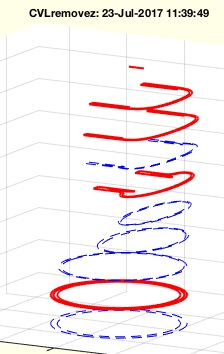% CVLremovez(CVL,z) - removes contours with a defined z value from a CVL% (by Tim Lueth, VLFL-Lib, 2017-JUL-23 as class: CLOSED POLYGON LISTS)% % Introduced first in SolidGeometry 4.0% % See also: CVLseparatez% % XVL=CVLremovez(CVL,z)% === INPUT PARAMETERS ===% CVL: Contour vertex list% z: list of z values to remove% === OUTPUT RESULTS ======% XVL: New Contour vertex list% % EXAMPLE: % load JACO_robot.mat; CVL=CVLofSGslices(JC0,10);% z=CVLseparatez(CVL)% CVLremovez(CVL,z([1 3 4 5 7]))% % See also: CVLseparatez% % % Copyright 2017 Tim C. Lueth
###### isintegerbv(x)- returns whether a number is an integer by value not by type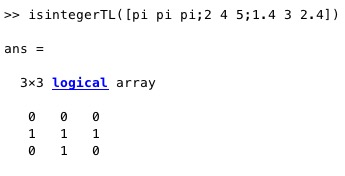% isintegerbv(x) - returns whether a number is an integer by value not by type% (by Tim Lueth, VLFL-Lib, 2017-JUL-20 as class: AUXILIARY PROCEDURES)% % mod(x,1)==0% % (Status of: 2017-07-21)% % Introduced first in SolidGeometry 4.0% % See also: isinteger% % l=isintegerbv(x)% === INPUT PARAMETERS ===% x: scalar, array or vector% === OUTPUT RESULTS ======% l: logical value,array or vector% % EXAMPLE: % isintegerTL([pi pi pi;2 4 5;1.4 3 2.4])% % See also: isinteger% % % Copyright 2017 Tim C. Lueth
###### meshgridofBB(SG,nx,ny,nz,thr)- returns a meshgrid for the coordinates of a solid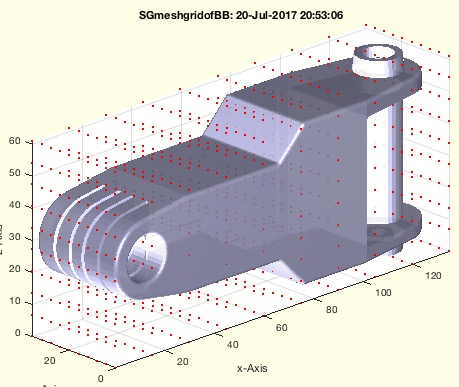% meshgridofBB(SG,nx,ny,nz,thr) - returns a meshgrid for the coordinates of a solid% (by Tim Lueth, VLFL-Lib, 2017-JUL-20 as class: SURFACES)% % Introduced first in SolidGeometry 4.0% % See also: VLmeshgrid% % [X,Y,Z,VL,xx,yy,zz]=meshgridofBB(SG,[nx,ny,nz,thr])% === INPUT PARAMETERS ===% SG: Solid geometry% nx: number of steps in x-direction% ny: number of steps in y-direction% nz: number of steps in z-direction% thr: elongation before and after; default is 0% === OUTPUT RESULTS ======% X: X-Values of Meshgrid% Y: Y-Values of Meshgrid% Z: Z-Values of Meshgrid% VL: Vertex List of the meshgrid% xx: steps in x-direction% yy: steps in y-direction% zz: steps in z-direction% % EXAMPLE: % SG=SGreadSTL(which('ForearmLink.stl'))% [X,Y,Z]=meshgridofBB(SG,10,10,10)% % [~,~,~,VL]=meshgridofBB(SG,20,20,20); SGisInterior(SG,VL);% % See also: VLmeshgrid% % % Copyright 2017 Tim C. Lueth
###### pdeplotfaces(model)- simply plots the surfaces to select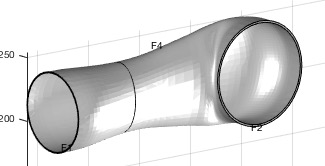% pdeplotfaces(model) - simply plots the surfaces to select% (by Tim Lueth, VLFL-Lib, 2017-JUL-20 as class: FEM/PDE)% % Introduced first in SolidGeometry 4.0% % See also: pdemodelofSG% % pdeplotfaces(model)% === INPUT PARAMETERS ===% model: pde model% % EXAMPLE: % % model=pdemodelofSG(SG);% pdeplotfaces(model);% % See also: pdemodelofSG% % % Copyright 2017 Tim C. Lueth
###### pdeplotresult(model,result)- plots the displacement in 3 quadrants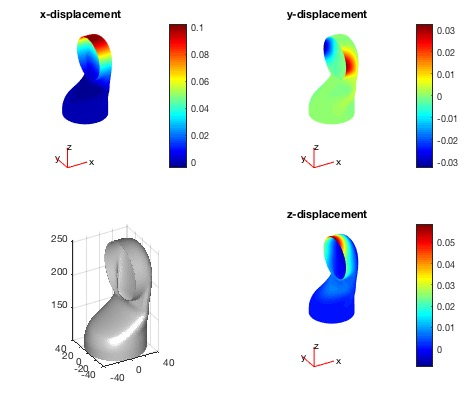% pdeplotresult(model,result) - plots the displacement in 3 quadrants% (by Tim Lueth, VLFL-Lib, 2017-JUL-20 as class: FEM/PDE)% % This fnctn is a plotting fnctn for showing the displacement results % under load (Status of: 2018-02-28)% % Introduced first in SolidGeometry 4.0% % See also: pdemodelofSG, pdeplotfaces, pdesolvesurfaceload, % pdestressstatic% % hf=pdeplotresult(model,result)% === INPUT PARAMETERS ===% model: pde model created by pdemodelofSG% result: pde result created by pdesolvesurfaceload% === OUTPUT RESULTS ======% hf: handle to figure% % EXAMPLE: Displacement of a robot link% load JACO_robot.mat% SG=SGseparate(JC1,6)% model=pdemodelofSG(SG)% pdesolvesurfaceload(model,1,2,[1 0 0]*1e4)% % See also: pdemodelofSG, pdeplotfaces, pdesolvesurfaceload, % pdestressstatic% % % Copyright 2017-2018 Tim C. Lueth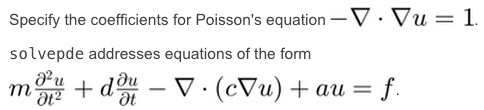% pdesolvesurfaceload(model,fixfacet,loadfacet,loadvec) - calculates the FEM analysis using pde for a pde mesh model% (by Tim Lueth, VLFL-Lib, 2017-JUL-19 as class: FEM/PDE)% % search also at matlab central for % von-mises-effective-stress-and-displacements !!! (Status of: 2018-02-28)% % Introduced first in SolidGeometry 4.0% % See also: pdemodelofSG, pdeplotfaces, pdeplotresult, pdestressstatic% % % [result,model]=pdesolvesurfaceload(model,[fixfacet,loadfacet,loadvec])% === INPUT PARAMETERS ===% model: pdemodel created by pdemodelofSG% fixfacet: list of facet that are fixed% loadfacet: list of faces that are loaded% loadvec: direction of load% === PROPERTY NAMES =====% 'FixedFaceIndices' : list of facet that are fixed% 'LoadFaceIndices' : list of faces that are loaded% 'Load' : direction of load% === OUTPUT RESULTS ======% result: simulation result% model: simulated model; called by reference!% % EXAMPLE: Displacement of a bracket with hole% A=SGreadSTL(which('BracketWithHole.stl'),1000)% SGfigure; FSplot(A), view(30,30)% model=pdemodelofSG(A)% pdesolvesurfaceload(model,3,9,1e3)% % pdesolvesurfaceload(model,' FixedFaceIndices',3,' LoadFaceIndices',9,' % Load',1e3)% % See also: pdemodelofSG, pdeplotfaces, pdeplotresult, pdestressstatic% % % % Copyright 2017-2018 Tim C. Lueth
###### pdemodelofSG(SG,h,e)- creates a pde model from a solid geometry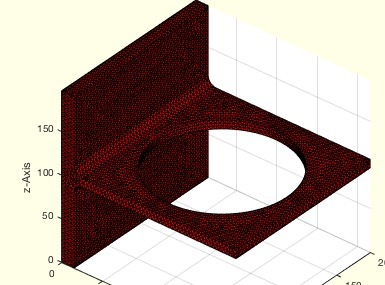% pdemodelofSG(SG,h,e) - creates a pde model from a solid geometry% (by Tim Lueth, VLFL-Lib, 2017-JUL-19 as class: FEM/PDE)% % This fnctn creates a pde model from a given solid. The pde models are % typically loaded from a STL File similar to Simccape Multibody. % Therefor this fnctn writes a temp solid and reads it afterwards. % % A pde model consists of a tetrahedral cross-linking (there are several % possibilities for the fnctn generateMesh), while one, two or % three-dimensional propagation of effects can be specified (line % problems, surface deformation, volume deformation) The standard FEM % equation will be specified. For these effects, the equilibrium % condition formula can be selected or the parameters for the standard % FEM equation specified. Subsequently, surfaces / introduction points % with state specifications are given and surfaces / initiation point are % given the effects of the action.% % ATTENTION: models are objects and modified by MATLAB fnctn with calls % by reference! It means the models are modified by a call even the % models are not an output parameter! The repeated call of % applyBoundaryCondition(model, .... % will add at each time conditions to the model even if the conditions % are all the same!% % *MOTIVATION FOR THE PDE fnctns IN SG-LIB* The Partial Diffential % Equation Toolbox (pde) contains some features that are helpful for the % basic processing of solids. However, some of this feature does not have % direct access, such as patching. Since the user can only access the % faces assignment via a graphical representation, the SG-Lib has to % implement a similar faces seperation. The same applies to the % production of the mesh tesselation. (Status of: 2018-02-28)% % Introduced first in SolidGeometry 4.0% % See also: pdemodelofSG, SGtetramesh, pdeplotfaces, pdesolvesurfaceload, % pdeplotresult, pdestressstatic% % [model,SG]=pdemodelofSG(SG,[h,e])% === INPUT PARAMETERS ===% SG: Solid Geoemtry% h: Hmax parameter for generateMesh% % e: e modulus default is 3000Pa = 3e9% === OUTPUT RESULTS ======% model: pde model for pde tool% SG: % % EXAMPLE: Deforming a Bracket:% A=SGreadSTL(which('BracketWithHole.stl'),1000)% model=pdemodelofSG(A)% pdeplot3D(model)% % A=SGsample(27)% model=pdemodelofSG(A)% SGfigure; pdeplot3D(model)% % See also: pdemodelofSG, SGtetramesh, pdeplotfaces, pdesolvesurfaceload, % pdeplotresult, pdestressstatic% % % Copyright 2017-2018 Tim C. Lueth
###### SGtetramesh(SG,h)- creates a tetramesh of a Solid Geometry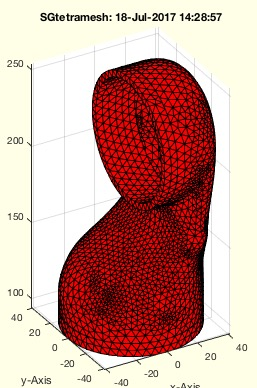% SGtetramesh(SG,h) - creates a tetramesh of a Solid Geometry% (by Tim Lueth, VLFL-Lib, 2017-JUL-18 as class: FEM/PDE)% % This fnctn uses the importGeometry and generateMesh fnctns of the % PDE-Toolbox to create a mesh of the solid an creates a triangualatin % from this data.% ATTENTION: SGtetramesh WILL NOT MELT SEPERATED SOLIDS, They are still % independent surfaces% In contrast to the normal PDE mesh, the linear mode is used here to % create the 4-facet meshes directly% % If SGtetramesh fails with error: "The input mesh is invalid" => % SGcheckmeshlab% If SGtetramesh fails with error: "Meshing failed with a Hmax of" => % Self intrusion! (Status of: 2018-08-24)% % Introduced first in SolidGeometry 4.0% % See also: pdemodelofSG% % [SGn,VL,FL,TR]=SGtetramesh(SG,[h])% === INPUT PARAMETERS ===% SG: Solid Geoemtry% h: maximum mesh length; default is sofBB/20% === OUTPUT RESULTS ======% SGn: Solid Geoemtry based on a PDE mesh% VL: Vertex list% FL: Facet list% TR: Tetrahedron triangulation% % EXAMPLE: % SGtetramesh(SGsample(17))% SGtetramesh(SGsample(17),3)% SGtetramesh(SGsample(35)) % failes because of non manifold% SGtetramesh(SGsample(36)) % failes because of self intrusion% % See also: pdemodelofSG% % % Copyright 2017-2018 Tim C. Lueth
###### exp_2017_07_18 (CVLz)- EXPERIMENT for automatic generation of packaging for filling from above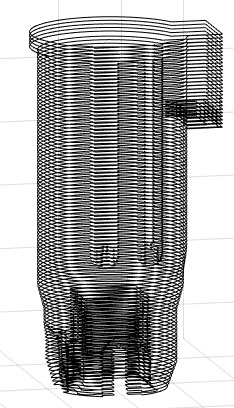% exp_2017_07_18 (CVLz) - EXPERIMENT for automatic generation of packaging for filling from above% (by Tim Lueth, VLFL-Lib, 2017-JUL-18 as class: EXPERIMENTS)% % Automatic generation of packaging for filling from above (z-axis) % (Status of: 2017-07-20)% % Introduced first in SolidGeometry 4.0% % exp_2017_07_18(CVLz)% === INPUT PARAMETERS ===% CVLz: % % % Copyright 2017 Tim C. Lueth
###### CVLofVL(CVL)- converts a CVL in a CVL whose contours are closed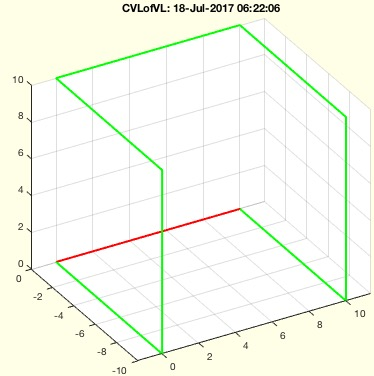% CVLofVL(CVL) - converts a CVL in a CVL whose contours are closed% (by Tim Lueth, VLFL-Lib, 2017-JUL-18 as class: CLOSED POLYGON LISTS)% % Introduced first in SolidGeometry 4.0% % See also: CPLofPL% % CVL=CVLofVL(CVL)% === INPUT PARAMETERS ===% CVL: CVL with potential open contours% === OUTPUT RESULTS ======% CVL: CVL with ensured closed contours% % EXAMPLE: % CVLofVL(VLsample(14))% % See also: CPLofPL% % % Copyright 2017 Tim C. Lueth
###### SGhollowsolid(SG,wthk,dist)- returns a copy of the solid with thin walls at an optional distance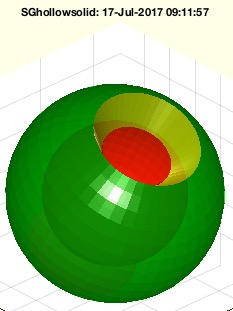% SGhollowsolid(SG,wthk,dist) - returns a copy of the solid with thin walls at an optional distance% (by Tim Lueth, VLFL-Lib, 2017-JUL-16 as class: SURFACES)% % *Hollow structures from closed solid surface models:* For all closed % surfaces it is easy: Create a grown/shrinked copy of the solid with a % defined wall thickness. For grown copies (shells), invert afterwards % the inner/original faces of the solid. for shrinked copies (hollow % solid walls), invert afterwards the inner/shrinked faces of the copy. % Both surfaces together are the final hollow solid.% ATTENTION: For a closed surface for most of the 3D printing processes % it makes no sense to convert solids into shell structures since the % material in the shells is not removable. There is no weight advantage!% The vertices are sorted: VL=[ORG;COPY]; The facets are sorted % FL=[ORG;COPY]% % *Solid structures from open free form surfaces:* In case of open % surfaces that do not represent a solid, this fnctn will create also % create a shrinked or grown copy and calculates in addition the facets % that link the corresponding walls.% The vertices are sorted: VL=[ORG;COPY]; The facets are sorted % FL=[ORG;COPY,WALL]% % *PRINCIPLE* Since the normal vector of all vertices is known, the grow % procedure will move this vertices along the normal vector. For an % isolated surface the normal vector can also be calculated, but in case % of features edges or features surfaces, the normal vector of the edge % contour cannot be calculated isolated from other attached surfaces.% % *ERROR CONDITIONS* In case that a isolated surface is processed, the % grow fnctn will not work as expected. This fnctn is for processing % connected surfaces of a closed solid only% (Status of: 2018-08-20)% % Introduced first in SolidGeometry 4.0% % See also: isplanarVLFL(VL, FL), VLFLsample, vertexNormalTL% % NSG=SGhollowsolid([SG,wthk,dist])% === INPUT PARAMETERS ===% SG: Solid Geometry% wthk: Wall thickness% dist: distance between original and new solid% === OUTPUT RESULTS ======% NSG: Copy with thin walls% % EXAMPLE: % A=SGsample(41); SGpuzzlecut3D(SGhollowsolid(A,0.7,.3));B=ans; SGplot(A,'w');% % [SG,FLW]=SGsphere(10,'',pi/3,-pi/3); SG.FL=FLW; % SG=SGtransR(SG,rot(pi/6,0,0)); SGfigure(SG); view(-30,30); VL=SG.VL; FL=SG.FL;% % [SG]=SGsphere(10,'',pi/3,-pi/3); % SGhollowsolid(SG,5)% % [VL,FL,SG]=VLFLsample(3); SGhollowsolid(SG,5)% % See also: isplanarVLFL(VL, FL), VLFLsample, vertexNormalTL% % % Copyright 2017-2018 Tim C. Lueth
###### VLFLsample(Nr,T)- returns OPEN surfaces as samples for procedure testing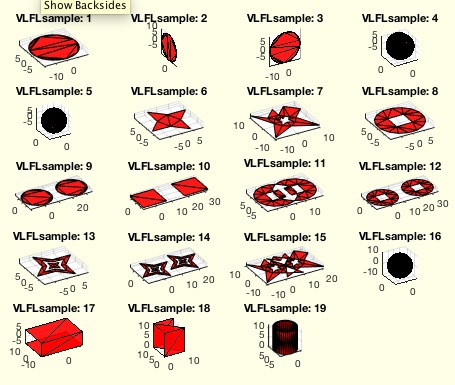% VLFLsample(Nr,T) - returns OPEN surfaces as samples for procedure testing% (by Tim Lueth, VLFL-Lib, 2017-JUL-16 as class: SURFACES)% % Introduced first in SolidGeometry 4.0% % See also: CPLsample, SGsample, VLsample, PLsample, CSGsample, % SGerrorsample, testfunctTL, permutevector% % [VL,FL,SG,CVL]=VLFLsample([Nr,T])% === INPUT PARAMETERS ===% Nr: Number of sample% T: Optional spatian transformation% === OUTPUT RESULTS ======% VL: Vertex List% FL: Facet List% SG: Solid SG.VL,SG.FL% CVL: Open Edge contour% % EXAMPLE: % VLFLsample(13)% VLFLsample(13,TofR(rot(pi/6,pi/6,0),[0 0 10]))% [~,~,~,CVL]=VLFLsample(13); SGfigure; CVLplot(CVL,'-',2); view(-30,30)% % See also: CPLsample, SGsample, VLsample, PLsample, CSGsample, % SGerrorsample, testfunctTL, permutevector% % % Copyright 2017-2018 Tim C. Lueth
###### SGnegativeform(SG,w)- creates from a solid a negative form with specific features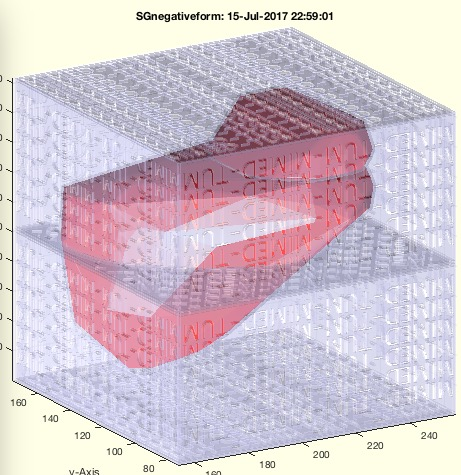% SGnegativeform(SG,w) - creates from a solid a negative form with specific features% (by Tim Lueth, VLFL-Lib, 2017-JUL-15 as class: SURFACES)% % Introduced first in SolidGeometry 4.0% % See also: SGconvexHull% % SG=SGnegativeform(SG,[w])% === INPUT PARAMETERS ===% SG: Solid Geometry% w: wall distance% === PROPERTY NAMES =====% 'Faces' : reduces the number of facets% 'turn' : if 'turn' is used, the axis are changed% 'convex' : if 'convex' is used, the convex hull is calculated% 'Type' : 'box' or 'shell' is supported; default is box% 'Cut' : cutting planes; default is uncut, i.e. [1 1 1]% 'hollowcontour' : % 'hollow' : % === OUTPUT RESULTS ======% SG: A simple negative for or several parts of a form% % See also: SGconvexHull% % % Copyright 2017 Tim C. Lueth
###### exp_2017_07_14- EXPERIMENT for creating a negative Form of a solid and perforate the shell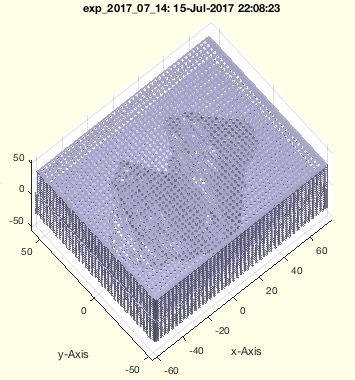% exp_2017_07_14 - EXPERIMENT for creating a negative Form of a solid and perforate the shell% (by Tim Lueth, VLFL-Lib, 2017-JUL-14 as class: SURFACES)% % Tutorial to show the power of SG-Lib for medical data. Discussed with % Franz Irlinger and Dominik Rumschoettel (Status of: 2017-07-14)% % Introduced first in SolidGeometry 4.0% % See also: SGnegativeform% % exp_2017_07_14% % See also: SGnegativeform% % % Copyright 2017 Tim C. Lueth
###### SGTdimensioning(SG,Fr,pnt)- creates a four-quadrant view related to a frame or the center of a solid geometry% SGTdimensioning(SG,Fr,pnt) - creates a four-quadrant view related to a frame or the center of a solid geometry% (by Tim Lueth, VLFL-Lib, 2017-JUL-13 as class: ANALYTICAL GEOMETRY)% % Introduced first in SolidGeometry 4.0% % See also: viewdimensioning, PLdimensioning, CVLdimclassifier% % [CPL1,CPL2,CPL3]=SGTdimensioning(SG,[Fr,pnt])% === INPUT PARAMETERS ===% SG: Solid Geometry % Fr: Frame Name; default is 'B'% pnt: center of view related to Frame% === OUTPUT RESULTS ======% CPL1: CPL of XY-Plane: subplot(2,2,4)% CPL2: CPL of XZ-Plane: subplot(2,2,2)% CPL3: CPL of YZ-Plane: subplot(2,2,1)% % EXAMPLE: % SGTdimensioning(SGsample(5))% SGTdimensioning(SGsample(17),'',[10 -0 -10])% % See also: viewdimensioning, PLdimensioning, CVLdimclassifier% % % Copyright 2017 Tim C. Lueth
###### ellipseofPL(PL)- returns the parameter for an ellipse of at least 5 points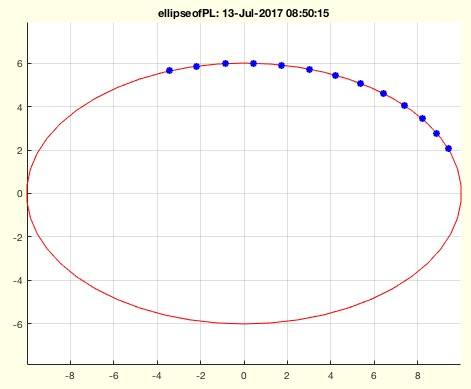% ellipseofPL(PL) - returns the parameter for an ellipse of at least 5 points% (by Ohad Gal, VLFL-Lib, 2017-JUL-13 as class: CLOSED POLYGON LISTS)% % Copyright (c) 2003, Ohad Gal% All rights reserved.% % Redistribution and use in source and binary forms, with or without % modification, are permitted provided that the following conditions are % met:% % * Redistributions of source code must retain the above copyright % notice, this list of conditions and the following disclaimer.% * Redistributions in binary form must reproduce the above copyright % notice, this list of conditions and the following disclaimer in the % documentation and/or other materials provided with the distribution% % THIS SOFTWARE IS PROVIDED BY THE COPYRIGHT HOLDERS AND CONTRIBUTORS "AS % IS" AND ANY EXPRESS OR IMPLIED WARRANTIES, INCLUDING, BUT NOT LIMITED % TO, THE IMPLIED WARRANTIES OF MERCHANTABILITY AND FITNESS FOR A % PARTICULAR PURPOSE ARE DISCLAIMED. IN NO EVENT SHALL THE COPYRIGHT % OWNER OR CONTRIBUTORS BE LIABLE FOR ANY DIRECT, INDIRECT, INCIDENTAL, % SPECIAL, EXEMPLARY, OR CONSEQUENTIAL DAMAGES (INCLUDING, BUT NOT % LIMITED TO, PROCUREMENT OF SUBSTITUTE GOODS OR SERVICES; LOSS OF USE, % DATA, OR PROFITS; OR BUSINESS INTERRUPTION) HOWEVER CAUSED AND ON ANY % THEORY OF LIABILITY, WHETHER IN CONTRACT, STRICT LIABILITY, OR TORT % (INCLUDING NEGLIGENCE OR OTHERWISE) ARISING IN ANY WAY OUT OF THE USE % OF THIS SOFTWARE, EVEN IF ADVISED OF THE POSSIBILITY OF SUCH DAMAGE.% (Status of: 2017-07-13)% % See also: center3P, center4P% % [cp,a,b,ex,ey,phi]=ellipseofPL(PL)% === INPUT PARAMETERS ===% PL: Point List% === OUTPUT RESULTS ======% cp: center % a: long axis% b: short axis% ex: ex vector% ey: ey vector% phi: turning angle% % EXAMPLE: % ellipseofPL(PLcircle(10,'',pi/2,6,pi/9))% % % See also: center3P, center4P%
###### exp_2017_07_13(CPL,n,s,noise);- EXPERIMENT to test ellipseofPL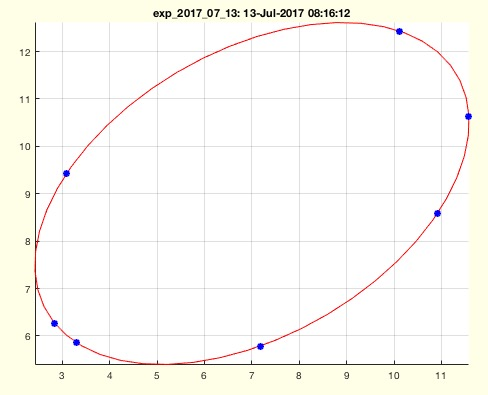% exp_2017_07_13(CPL,n,s,noise); - EXPERIMENT to test ellipseofPL% (by Tim Lueth, VLFL-Lib, 2017-JUL-13 as class: EXPERIMENTS)% % Introduced first in SolidGeometry 4.0% % See also: ellipseofPL% % cp=exp_2017_07_13([CPL,n,s,noise]);% === INPUT PARAMETERS ===% CPL: Close Polygon to test% n: number of points used for testing% s: starting index for a succeeding sequence; 0 = random% noise: noise added to the point list coordinates% === OUTPUT RESULTS ======% cp: % % See also: ellipseofPL% % % Copyright 2017 Tim C. Lueth
###### SGdimensioning(SG,ang1,ang2,pnt)- creates a drqwing of the solid including dimensions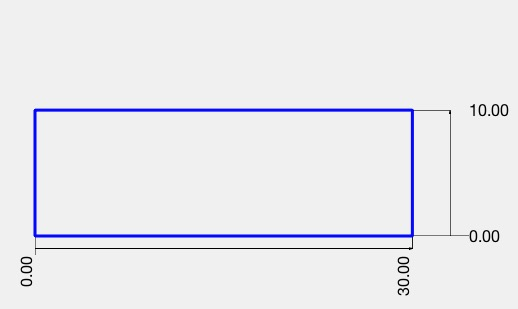% SGdimensioning(SG,ang1,ang2,pnt) - creates a drqwing of the solid including dimensions% (by Tim Lueth, VLFL-Lib, 2017-JUL-12 as class: SURFACES)% % Introduced first in SolidGeometry 4.0% % See also: SGTdimensioning, viewdimensioning, PLdimensioning, % CVLdimclassifier% % SGdimensioning(SG,[ang1,ang2,pnt])% === INPUT PARAMETERS ===% SG: Solid geometry% ang1: angle 1 similar to view% ang2: angle 2 similar to view% pnt: crossing point% % EXAMPLE: % A=SGbox([30,20,10])% SGdimensioning(A,0,0)% SGdimensioning(A,0,90)% SGdimensioning(A,90,0)% % See also: SGTdimensioning, viewdimensioning, PLdimensioning, % CVLdimclassifier% % % Copyright 2017 Tim C. Lueth
###### CVLofopenfaces(VL,FL,thr)- returns the closed vertex list for all open surfaces% CVLofopenfaces(VL,FL,thr) - returns the closed vertex list for all open surfaces% (by Tim Lueth, VLFL-Lib, 2017-JUL-12 as class: SURFACES)% % This fnctn is more or less for testing purposes. The fnctn % VLFLcorrectdoubledvertex is called first, since otherwise it makes no % sense to detect open surfaces (Status of: 2017-07-12)% % Introduced first in SolidGeometry 4.0% % See also: VLFLcorrectdoubledvertex% % % [CVL,EL,CIL]=CVLofopenfaces(VL,FL,[thr])% === INPUT PARAMETERS ===% VL: Vertex list% FL: Facet list% thr: threshold; default is 1e-3 for VLFLcorrectdoubledvertex% === OUTPUT RESULTS ======% CVL: Closed Vertex List% EL: Edge List% CIL: Contour Index List for EL% % EXAMPLE: % loadweb JACO_robot.mat; A=SGseparate(JC2,2)% CVLofopenfaces(A.VL,A.FL);% % CVL=CVLofopenfaces(A.VL,A.FL);% CVLdimclassifier(CVL)% % See also: VLFLcorrectdoubledvertex% % % % Copyright 2017 Tim C. Lueth
###### VLFLerroropenfaces(VL,FL)- creates an open faces error by adding an existing vertex of a random facet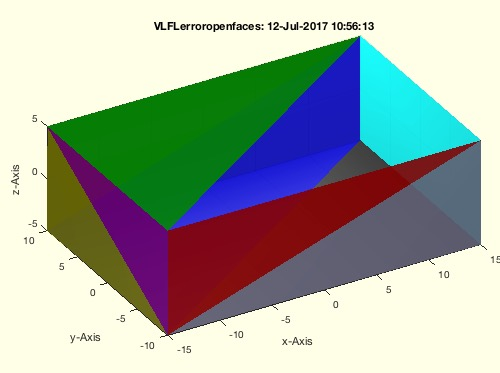% VLFLerroropenfaces(VL,FL) - creates an open faces error by adding an existing vertex of a random facet% (by Tim Lueth, VLFL-Lib, 2017-JUL-12 as class: AUXILIARY PROCEDURES)% % This fnctn deletes existing facets/surfaces and adds two degenerated % facets.% if the vertex list contains doubled vertices, the fnctn surfaceofSG is % unable to find the connected surfaces (Status of: 2017-07-12)% % Introduced first in SolidGeometry 4.0% % See also: VLFLcorrectdoubledvertex, VLFLerrordoubledvertex% % [VL,FL]=VLFLerroropenfaces(VL,FL)% === INPUT PARAMETERS ===% VL: Vertex List% FL: Facet List% === OUTPUT RESULTS ======% VL: Unchanged Vertex List% FL: Facet list with a surface missing% % EXAMPLE: % A=SGbox([30,20,10]); for i=1:4; % [A.VL,A.FL]=VLFLerrordoubledvertex(A.VL,A.FL); end% VLFLerroropenfaces(A.VL,A.FL)% % See also: VLFLcorrectdoubledvertex, VLFLerrordoubledvertex% % % Copyright 2017 Tim C. Lueth
###### VLFLcorrectdoubledvertex(VL,FL,thr)- finds and removes doubled vertex entries and shows degenerated facts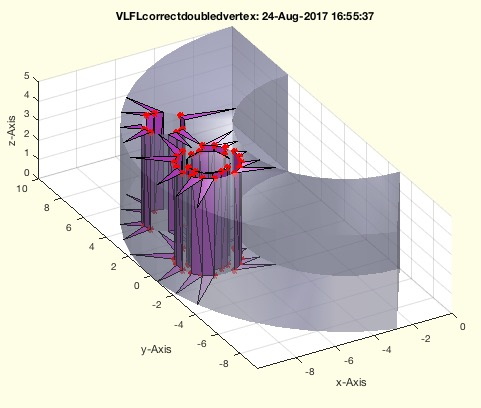% VLFLcorrectdoubledvertex(VL,FL,thr) - finds and removes doubled vertex entries and shows degenerated facts% (by Tim Lueth, VLFL-Lib, 2017-JUL-12 as class: AUXILIARY PROCEDURES)% % After removal of doubled vertices, there can be degenerated facets of % type [vi vk vk]. Those degenerated Facet MUST NOT BE REMOVED! (Status % of: 2017-08-24)% % Introduced first in SolidGeometry 4.0% % See also: VLFLerrordoubledvertex, VLFLerroropenfaces% % [VLN,FLN,n]=VLFLcorrectdoubledvertex(VL,FL,[thr])% === INPUT PARAMETERS ===% VL: Vertex list% FL: Facet list% thr: resolution; default is 1e-12% === OUTPUT RESULTS ======% VLN: Shortend certex list with original, not rounded vertex values% FLN: Corrected facet list% n: number of removed vertices% % EXAMPLE: % A=SGbox([30,30,1]); VLFLcorrectdoubledvertex(A.VL,A.FL,2);% A=SGsample(25); VLFLcorrectdoubledvertex(A.VL,A.FL,1);% A=SGbox([30,20,10])% [B.VL,B.FL]=VLFLerrordoubledvertex(A.VL,A.FL)% [C.VL,C.FL]=VLFLcorrectdoubledvertex(B.VL,B.FL)% % See also: VLFLerrordoubledvertex, VLFLerroropenfaces% % % Copyright 2017 Tim C. Lueth
###### VLFLerrordoubledvertex(VL,FL)- creates an doubled vertex error by adding an existing vertex of a random facet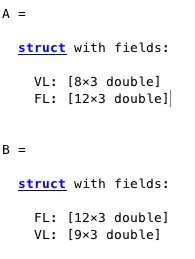% VLFLerrordoubledvertex(VL,FL) - creates an doubled vertex error by adding an existing vertex of a random facet% (by Tim Lueth, VLFL-Lib, 2017-JUL-12 as class: AUXILIARY PROCEDURES)% % Testing fnctn for development that creates copies of vertices plus a % noise. (Status of: 2017-07-12)% % Introduced first in SolidGeometry 4.0% % See also: VLFLcorrectdoubledvertex, VLFLerroropenfaces% % [VL,FL]=VLFLerrordoubledvertex(VL,FL)% === INPUT PARAMETERS ===% VL: Vertex List% FL: Facet List% === OUTPUT RESULTS ======% VL: vertex list with an additional/doubled entry% FL: facet list indexing the doubled entry% % EXAMPLE: % A=SGbox([30,20,10])% [B.VL,B.FL]=VLFLerrordoubledvertex(A.VL,A.FL)% [C.VL,C.FL]=VLFLcorrectdoubledvertex(B.VL,B.FL)% % See also: VLFLcorrectdoubledvertex, VLFLerroropenfaces% % % Copyright 2017 Tim C. Lueth
###### JACOmodel(gl,siz,tck);- creates an STL file for magnified/shrinked jaco link% JACOmodel(gl,siz,tck); - creates an STL file for magnified/shrinked jaco link % (by Tim Lueth, ROBOT-Lib, 2017-JUL-11 as class: FILE HANDLING)% % This fnctn is to created shrinked versions of the JACO STL's. Since the % STL file contain different closed and even some open surfaces, it is % required to select some of the parts to grow the to a required wall % thickness (Status of: 2017-07-13)% % Introduced first in SolidGeometry 4.0% % See also: JACOsim, JACOget, JACOset% % SGS=JACOmodel([gl,siz,tck]);% === INPUT PARAMETERS ===% gl: robot link number% siz: shinking/magnifiaction size; default is 0.25% tck: thickness addon; default is 1.0% === OUTPUT RESULTS ======% SGS: Final Solid including frames% % EXAMPLE: just type% JACOmodel% % See also: JACOsim, JACOget, JACOset% % % Copyright 2017 Tim C. Lueth
###### FSofSG(TR,alpha,sizesort,Amin)- Features surfaces of SG% FSofSG(TR,alpha,sizesort,Amin) - Features surfaces of SG% (by Tim Lueth, VLFL-Lib, 2017-JUL-09 as class: SURFACES)% % This fnctn can take several seconds to classify all OPEN surfaces of a % solid by using TR3neighborsAngle and neighborsAngleSurface. When called % without output parameter; drawing the surfaces take the most time. % (Status of: 2017-07-31)% % Introduced first in SolidGeometry 4.0% % See also: FSplot, surfacesofSG% % [CFL,nF,Ai]=FSofSG(TR,[alpha,sizesort,Amin])% === INPUT PARAMETERS ===% TR: Solid Geometry or Triangulation% alpha: maximum angle difference between two facets; default is 1 ~ 60 % degree% sizesort: if true, the surfaces are sorted by their size; default is % false% Amin: Minimum area to be classified as individual surface% === OUTPUT RESULTS ======% CFL: Index List for the Surface Indices% nF: maximum value of FIL% Ai: Facet Normal List - TR3neighborsAngle% % EXAMPLE: % load JACO_robot.mat% FSofSG(JC3,0.6);% FSofSG(JC3,0.6,true);% % See also: FSplot, surfacesofSG% % % Copyright 2017-2018 Tim C. Lueth
###### VLmeshgrid(xx,yy,zz)- simple function for a better understand of meshgrid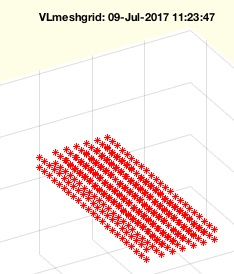% VLmeshgrid(xx,yy,zz) - simple fnctn for a better understand of meshgrid% (by Tim Lueth, VLFL-Lib, 2017-JUL-09 as class: AUXILIARY PROCEDURES)% % Introduced first in SolidGeometry 4.0% % See also: CPLofmeshgrid(X, Y), PLmeshgrid% % VL=VLmeshgrid(xx,yy,[zz])% === INPUT PARAMETERS ===% xx: list of x values% yy: list of y values% zz: list of y values; % === OUTPUT RESULTS ======% VL: Point list of all mesh coordinates% % EXAMPLE: % VLmeshgrid(1:3,1:2,10)% VLmeshgrid([0 15 25 35 45 55 65],0:8:8*22,[10 20])% % See also: CPLofmeshgrid(X, Y), PLmeshgrid% % % Copyright 2017 Tim C. Lueth
###### VLedgeNormal_old(VL,ez)- returns the normal vector to vertex edge list% VLedgeNormal_old(VL,ez) - returns the normal vector to vertex edge list% (by Tim Lueth, VLFL-Lib, 2017-JUL-09 as class: EDGE LISTS)% % =======================================================================% OBSOLETE (2017-07-26) - USE FAMILY 'VLedgeNormal' INSTEAD% =======================================================================% % It does not support nan separted vertex lists (CVL) yet. The normal is % NaN for the first point or the given ez. The normal is NaN for the % lastpoint. The normal is NaN for all straight points. In case of % switching normals; the orientation of the succeeding normal will be % fliped. (Status of: 2017-07-19)% % Introduced first in SolidGeometry 4.0% % See also: [ VLedgeNormal ] ; VLFLfaceNormal, VLedgeNormal, edgeNormal, % CPLedgeNormal% % [NL,AL]=VLedgeNormal_old(VL,[ez])% === INPUT PARAMETERS ===% VL: Vertex list of succeeding vertices% ez: optional first normal vector; default is normal of facet [1 2 3]% === OUTPUT RESULTS ======% NL: Normal vector; NaN if A(i)=0;% AL: area vector; if straight, A(i)=0;% % EXAMPLE: % VL=VLsample(12); VL=[VL;VL(1:2,:)]; VLedgeNormal(VL)% VLedgeNormal(PLhelix(10,10,4*pi))% VLedgeNormal(VLsample(9))% % % See also: [ VLedgeNormal ] ; VLFLfaceNormal, VLedgeNormal, edgeNormal, % CPLedgeNormal% % % Copyright 2017 Tim C. Lueth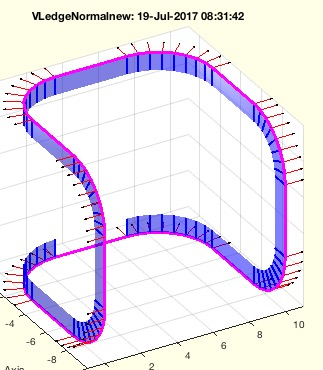% VLedgeNormal(VL,ez) - returs normal and plane vector for free boundary contour% (by Tim Lueth, VLFL-Lib, 2017-JUL-09 as class: CLOSED POLYGON LISTS)% % This fnctn is more complex to understand that it seems on the first % view: Three succeeding points [last current next] define a plane if not % in a line. This plane has a normal vector ez. The tangential vector % ex= is [next - last] and the plane vector is ey=cross(ez,ex)% 2018-07-23 Does also support straight lines now. (Status of: 2018-08-20)% % Introduced first in SolidGeometry 4.0% % See also: VLFLfaceNormal, VLFLofCVLdelaunay2D, VLFLofCVLdelaunay3D, % edgeNormal, CPLedgeNormal, Rofez% % [NL,OL]=VLedgeNormal(VL,[ez])% === INPUT PARAMETERS ===% VL: Vertex list of succeeding vertices% ez: optional first normal vector; default is normal of facet [1 2 3]% === OUTPUT RESULTS ======% NL: Normal Vector List (red)% OL: Orthogonal Plane Directed Vector list (blue)% % EXAMPLE: % VL=VLsample(12); VL=[VL;VL(1:2,:)]; VLedgeNormal(VL)% VLedgeNormal(PLhelix(10,10,4*pi))% VLedgeNormal(VLsample(9))% VLedgeNormal(VLsample(2))% % See also: VLFLfaceNormal, VLFLofCVLdelaunay2D, VLFLofCVLdelaunay3D, % edgeNormal, CPLedgeNormal, Rofez% % % Copyright 2017-2018 Tim C. Lueth
###### PLmeshgrid(xx,yy)- simple function for a better understand of meshgrid% PLmeshgrid(xx,yy) - simple fnctn for a better understand of meshgrid% (by Tim Lueth, VLFL-Lib, 2017-JUL-08 as class: AUXILIARY PROCEDURES)% % Introduced first in SolidGeometry 4.0% % See also: VLmeshgrid, CPLofmeshgrid(X, Y)% % PL=PLmeshgrid(xx,yy)% === INPUT PARAMETERS ===% xx: list of x values% yy: list of y values% === OUTPUT RESULTS ======% PL: Point list of all mesh coordinates% % EXAMPLE: % PLmeshgrid(1:3,1:2)% PLmeshgrid([0 15 25 35 45 55 65],0:8:8*22)% % See also: VLmeshgrid, CPLofmeshgrid(X, Y)% % % Copyright 2017 Tim C. Lueth
###### SGgrowFS (SG,t)- grows the feature surfaces of a solid% SGgrowFS (SG,t) - grows the feature surfaces of a solid% (by Tim Lueth, VLFL-Lib, 2017-JUL-08 as class: SURFACES)% % The feature surfaces are calculated (default value is 1 rad/60 degree). % Afterwards it is analyzed wich facets show inside of the solid an which % show outside. Only inside or outside are processed here. Use SGgrow for % processing all. At the border between outside and inside, the vertices % that belong to both (inside, outside) stay unchanged. The vertices that % define the border of the new outside surfaces are not moved along the % vertex normal vector but along the normal vector of the border contour!% Attention: Be aware that concave surface cannot be grown without % self-penetration. (Status of: 2017-07-08)% % Introduced first in SolidGeometry 4.0% % See also: SGgrowFS, SGgrow% % SGgrowFS(SG,[t])% === INPUT PARAMETERS ===% SG: Solid Geometry% t: Increase/Decrease Value along the vertex normal vector% % EXAMPLE: % load JACO_robot.mat % use loadweb if the file need to be aquired% SG=SGseparate(JC3,3)% SGgrowFS(SG,10)% % See also: SGgrowFS, SGgrow% % % Copyright 2017 Tim C. Lueth
###### clipboardrem(maxc)- removes comment characters from the clipboard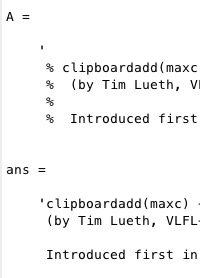% clipboardrem(maxc) - removes comment characters from the clipboard% (by Tim Lueth, FileMaker, 2017-JUL-07 as class: AUXILIARY PROCEDURES)% % Introduced first in SolidGeometry 4.0% % See also: clipboardadd% % C=clipboardrem([maxc])% === INPUT PARAMETERS ===% maxc: % === OUTPUT RESULTS ======% C: % % See also: clipboardadd% % % Copyright 2017 Tim C. Lueth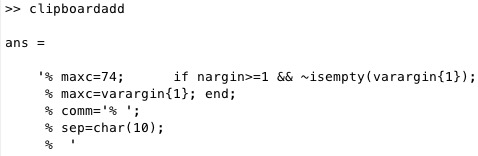% clipboardadd(maxc) - adds comment characters from the clipboard% (by Tim Lueth, VLFL-Lib, 2017-JUL-07 as class: AUXILIARY PROCEDURES)% % Introduced first in SolidGeometry 4.0% % See also: clipboardrem% % C=clipboardadd([maxc])% === INPUT PARAMETERS ===% maxc: % === OUTPUT RESULTS ======% C: % % See also: clipboardrem% % % Copyright 2017 Tim C. Lueth
###### surfacesofSG(TR,alpha)- returns all feature surfaces (open boundaries) that have a limited angle between their normal vectors% surfacesofSG(TR,alpha) - returns all feature surfaces (open boundaries) that have a limited angle between their normal vectors% (by Tim Lueth, VLFL-Lib, 2017-JUL-06 as class: SURFACES)% % This fnctn can take several seconds to classify all OPEN surfaces of a % solid by using TR3neighborsAngle and neighborsAngleSurface.% When called without output parameter; drawing the surfaces take the % most time.% SGsurfaces returns CLOSED surfaces% TR3mountingfaces is a very similar fnctn% (Status of: 2017-07-27)% % Introduced first in SolidGeometry 4.0% % See also: neighborsAngleSurface, TR3neighborsAngle, SGsurfaces, % TR3mountingfaces% % [FIL,k,FNL,NAL,NBL,Ai]=surfacesofSG(TR,[alpha])% === INPUT PARAMETERS ===% TR: Solid Geometry or Triangulation% alpha: maximum angle between two facets; default is 1 ~ 60 degree% === OUTPUT RESULTS ======% FIL: Index List for the Surface Indices% k: maximum value of FIL% FNL: Facet Normal List - TR3neighborsAngle% NAL: Neigbor Angle List - TR3neighborsAngle% NBL: Neigbor List - TR3neighborsAngle% Ai: Area of the indexed surfaces 1:k% % EXAMPLE: % load JACO_robot.mat% surfacesofSG(JC3);% % See also: neighborsAngleSurface, TR3neighborsAngle, SGsurfaces, % TR3mountingfaces% % % Copyright 2017 Tim C. Lueth
###### LaserInit- Initializes the USB-Serial Port and Requests Data from the Leuze Scanner% LaserInit - Initializes the USB-Serial Port and Requests Data from the Leuze Scanner% (by Tim Lueth & Sebastian Heininger, ROBOT-Lib, 2017-JUL-06)% % This is just an example how to use the Leuze Laser Scanner at the mac % computer. (Status of: 2017-07-06)% % Introduced first in SolidGeometry 4.0% % See also: USBhelp% % LaserInit% % See also: USBhelp% % % Copyright 2017 Tim C. Lueth
###### SGinsideSurface(SG,fnum,wlim,ilim)- Checks the distance between the centers of the facets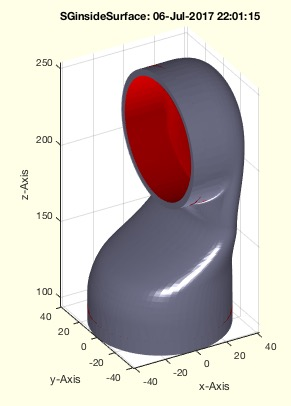% SGinsideSurface(SG,fnum,wlim,ilim) - Checks the distance between the centers of the facets% (by Tim Lueth, VLFL-Lib, 2017-JUL-06 as class: EXPERIMENTS)% % The fnctns SGcheckfacedist and SGinsidesurface are very similar. Both % ray trace the normal vector of a facet and detect self-penetration of % other facets. While SGcheckfacedist looks backwards, SGinsideSurface % looks forward along the normal vector. The only problem is the extreme % time-consuming quadratic growing effort. % In SGcheckfacedist there is almost never a need to process the original % data. But for SGinsidesurface there is wish to process the original % data. There we use a heuristics to accelerate the algorithm. First we % analyze freeeform surfaces using surfacesofSG, the we analyze just for % each of those surfaces ONE surface to have an opponent. (Status of: % 2017-07-07)% % Introduced first in SolidGeometry 4.0% % See also: SGcheckfacedist, SGinsidesurface, surfacesofSG% % [FIL,insFS]=SGinsideSurface(SG,[fnum,wlim,ilim])% === INPUT PARAMETERS ===% SG: Solid Geometry% fnum: reduce to face numbers; default is 3000;% wlim: warning limit; default is 0.7 mm% ilim: intended limit; distance that is considered as intended; default % is 0.1mm% === OUTPUT RESULTS ======% FIL: Facet surface index list% insFS: inside index of feature surface% % EXAMPLE: % load JACO_robot.mat% SG=SGsurfaces(JC3,3)% SGinsideSurface(SG)% % See also: SGcheckfacedist, SGinsidesurface, surfacesofSG% % % Copyright 2017 Tim C. Lueth
###### diffangle(NL1,NL2,normfirst)- calculates the angle between to normal vector lists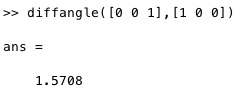% diffangle(NL1,NL2,normfirst) - calculates the angle between to normal vector lists% (by Tim Lueth, VLFL-Lib, 2017-JUL-06 as class: ANALYTICAL GEOMETRY)% % optimal for list processing. if normalization is required, the time is % about doubled (Status of: 2017-08-03)% % Introduced first in SolidGeometry 4.0% % See also: TR3neighborsAngle, PLangle, VLangle, PLangle2% % AL=diffangle(NL1,NL2,[normfirst])% === INPUT PARAMETERS ===% NL1: normal vector lists 1% NL2: normal vector lists 2% normfirst: if true; VLnorm is sued to norm the vectors first; % === OUTPUT RESULTS ======% AL: real(acos(dot(NL1(i,:),NL2(i,1))))% % EXAMPLE: NL1=rand(10,3); NL2=rand(10,3);% % % See also: TR3neighborsAngle, PLangle, VLangle, PLangle2% % % Copyright 2017 Tim C. Lueth
###### SGcheckfacedist(SG,fnum,wlim,ilim)- Checks the distance between the centers of the facets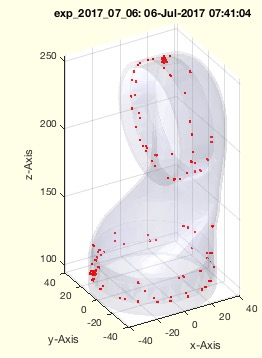% SGcheckfacedist(SG,fnum,wlim,ilim) - Checks the distance between the centers of the facets% (by Tim Lueth, VLFL-Lib, 2017-JUL-06 as class: EXPERIMENTS)% % Important test procedure for shrinked models and imported geometries. % Attention: Some geometries consist of intended overlapping structures % Attention: Some geometries consist of open surfaces (Status of: % 2017-07-06)% % Introduced first in SolidGeometry 4.0% % See also: exp_2017_07_06% % [ml,cpl]=SGcheckfacedist(SG,[fnum,wlim,ilim])% === INPUT PARAMETERS ===% SG: Solid Geometry% fnum: reduce to face numbers; default is 3000;% wlim: warning limit; default is 0.7 mm% ilim: intended limit; distance that is considered as intended; default % is 0.1mm% === OUTPUT RESULTS ======% ml: complete list of distances% cpl: complete list of cross points% % EXAMPLE: % load JACO_robot.mat% X=SGseparate(JC2,5)% SGcheckfacedist(X,2000)% % See also: exp_2017_07_06% % % Copyright 2017 Tim C. Lueth
###### VLFLofSGT(SGN,N)- returns the VL and FL of the surface related to a fram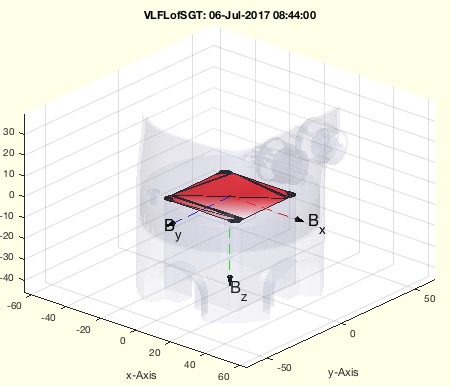% VLFLofSGT(SGN,N) - returns the VL and FL of the surface related to a fram% (by Tim Lueth, VLFL-Lib, 2017-JUL-06 as class: SURFACES)% % Introduced first in SolidGeometry 4.0% % See also: CVLofVLFL% % [VL,FL]=VLFLofSGT(SGN,N)% === INPUT PARAMETERS ===% SGN: Solid with Frames% N: Name of Frames% === OUTPUT RESULTS ======% VL: Vertex list; shortend% FL: FAcet list; shortend% % EXAMPLE: % loadweb JACO_robot.mat% VLFLofSGT(JC0,'B');% % See also: CVLofVLFL% % % Copyright 2017 Tim C. Lueth
###### CVLofVLFL()- does the same as CVLfreeBoundaryVLFL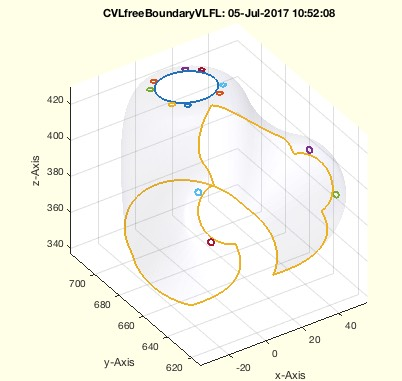% CVLofVLFL() - does the same as CVLfreeBoundaryVLFL% (by Tim Lueth, VLFL-Lib, 2017-JUL-06 as class: SURFACES)% % =======================================================================% OBSOLETE (2017-07-26) - USE FAMILY 'CVLfreeBoundaryVLFL' INSTEAD% =======================================================================% % Introduced first in SolidGeometry 4.0% % See also: [ CVLfreeBoundaryVLFL ] ; VLFLofSGT, CVLfreeBoundaryVLFL% % [CVL,TR]=CVLofVLFL([])% === OUTPUT RESULTS ======% CVL: % TR: % % See also: [ CVLfreeBoundaryVLFL ] ; VLFLofSGT, CVLfreeBoundaryVLFL% % % Copyright 2017 Tim C. Lueth
###### CVLofSGT(SGN,N)- returns the CVL of the surface that belongs to a Frame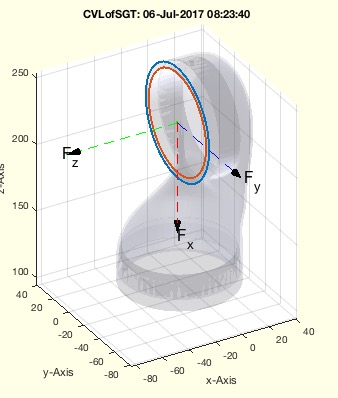% CVLofSGT(SGN,N) - returns the CVL of the surface that belongs to a Frame% (by Tim Lueth, VLFL-Lib, 2017-JUL-06 as class: SURFACES)% % currently required that the affiliated surfaces are defined already and % linked to the frame name. (Status of: 2017-08-11)% % Introduced first in SolidGeometry 4.0% % See also: VLFLofSGT, CVLofSGcutT, CVLofSGcutplanes% % [CVL,CPL]=CVLofSGT(SGN,N)% === INPUT PARAMETERS ===% SGN: Solid Geometry with Frames% N: Frame name or Transfomraton Matrx [4x4]% === OUTPUT RESULTS ======% CVL: Convtour Vertex List% CPL: Closed contour wrt Frame N; [n x 3]; z should be 0% % EXAMPLE: % loadweb JACO_robot.mat% CVLofSGT(JC1,'F');% CVLofSGT(JC1,TofP([0 0 150]));% % See also: VLFLofSGT, CVLofSGcutT, CVLofSGcutplanes% % % Copyright 2017 Tim C. Lueth
###### SGofsmbFullModelSimulation(SGi,Ti,tm,t)- returns a solid geometry for an arbitrary time value of a simulation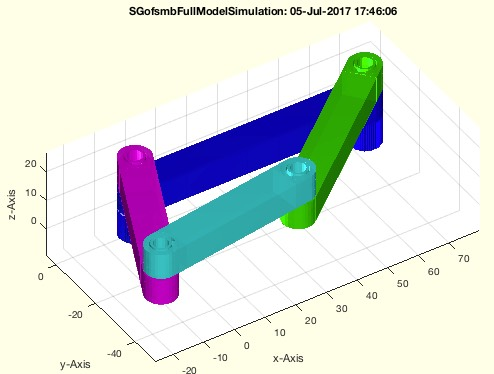% SGofsmbFullModelSimulation(SGi,Ti,tm,t) - returns a solid geometry for an arbitrary time value of a simulation% (by Tim Lueth, VLFL-Lib, 2017-JUL-05 as class: SIMMECHANICS INTERFACE)% % This fnctn is an add on to smbFullModelSimulation (Status of: % 2017-07-05)% % Introduced first in SolidGeometry 4.0% % See also: smbFullModelSimulation% % [SG,t]=SGofsmbFullModelSimulation(SGi,Ti,tm,t)% === INPUT PARAMETERS ===% SGi: List of Solid Geometries, result of smbFullModelSimulation% Ti: List of HT Frames, result of smbFullModelSimulation% tm: List of time points result of smbFullModelSimulation% t: desired time% === OUTPUT RESULTS ======% SG: Solid Geoemtry% t: Exact time of simulation% % EXAMPLE: % [~,BNi,SGi,Ti,tm]=smbFullModelSimulation(1);% SGofsmbFullModelSimulation(SGi,Ti,tm,0);% % See also: smbFullModelSimulation% % % Copyright 2017 Tim C. Lueth
###### SGgrabcad(page)- Simply opens the WWW-page of grabcad.com in the system browser% SGgrabcad(page) - Simply opens the WWW-page of grabcad.com in the system browser% (by Tim Lueth, VLFL-Lib, 2017-JUL-05 as class: AUXILIARY PROCEDURES)% % Introduced first in SolidGeometry 4.0% % See also: SG_DBconnectors.mat, SG_Patientknee.mat, DeepL% % SGgrabcad([page])% === INPUT PARAMETERS ===% page: optional direct page such as 'hebi'% % EXAMPLE: % SGgrabcad% SGgrabcad('hebi')% % See also: SG_DBconnectors.mat, SG_Patientknee.mat, DeepL% % % Copyright 2017-2018 Tim C. Lueth
###### CVLfreeBoundaryVLFL(VL,FL)- returns the CVL of the free boundary% CVLfreeBoundaryVLFL(VL,FL) - returns the CVL of the free boundary% (by Tim Lueth, VLFL-Lib, 2017-JUL-05 as class: SURFACES)% % Introduced first in SolidGeometry 4.0% % See also: freeBoundary, neighborsAngleSurface% % [CVL,TR2]=CVLfreeBoundaryVLFL(VL,FL)% === INPUT PARAMETERS ===% VL: Vertex List, or Solid Geometry, or triangulation% FL: Facet list, if VL is Vertex list% === OUTPUT RESULTS ======% CVL: Closed vertex contour list% TR2: triangulation% % EXAMPLE: % loadweb JACO_robot.mat% SG=JC61; [NAL,NBL]=TR3neighborsAngle(SG); % fi=neighborsAngleSurface(NAL,NBL,1,22000);% CVL=CVLfreeBoundaryVLFL(SG.VL,SG.FL(fi,:)); SGfigure; % CVLplot(CVL,'--'); % CV=separateNaN(CVL,10); CVLplot(CV,'-',3);% % See also: freeBoundary, neighborsAngleSurface% % % Copyright 2017 Tim C. Lueth
###### ELunique(ELo,remeq)- returns a unique list of all edges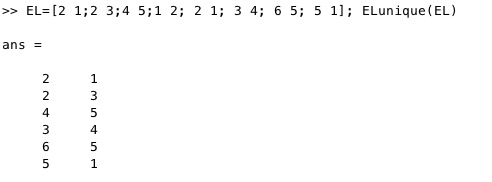% ELunique(ELo,remeq) - returns a unique list of all edges% (by Tim Lueth, VLFL-Lib, 2017-JUL-05 as class: EDGE LISTS)% % Makes sure that no edge is used twice!% More or less the same as ELuniqueofFL but will not change the ordner % and in case of doubles the direction of the first used edges is not % changed. (Status of: 2017-08-17)% % Introduced first in SolidGeometry 4.0% % See also: ELuniqueofFL, FLunique% % EL=ELunique(ELo,[remeq])% === INPUT PARAMETERS ===% ELo: Edge List or Facet List% remeq: removes degenerated edges [i i]; default is false;% === OUTPUT RESULTS ======% EL: Edge list sorted by first vertex index% % EXAMPLE: % EL=[2 1;2 3;4 5;1 2; 2 1; 3 4; 6 5; 5 1]; ELunique(EL)% ELunique([4 4;4 5; 5 4; 6 4],true)% % See also: ELuniqueofFL, FLunique% % % Copyright 2017 Tim C. Lueth
###### graphofEL(EL,n)- creates an undirected graph model from an edge list% graphofEL(EL,n) - creates an undirected graph model from an edge list% (by Tim Lueth, VLFL-Lib, 2017-JUL-05 as class: EDGE LISTS)% % This is the first fnctn to analyze surface models using graph theory.% It is extremely helpful to analyze the structures by this abstraction % level.% MATLAB supports since 2015b % undirected "graphs" and% directed "digraphs" (Status of: 2017-09-10)% % Introduced first in SolidGeometry 4.0% % See also: featureEdges, ELplot% % G=graphofEL(EL,[n])% === INPUT PARAMETERS ===% EL: Edge list; must have unique entries!% n: optional number of vertices% === OUTPUT RESULTS ======% G: MATLAB's Graph struct using Edges and Nodes% % EXAMPLE: % EL=featureEdges(TR3ofSG(SGsample(25)),1)% graphofEL(EL)% graphofEL(featureEdges(TR3ofSG(SGsample(3)),1))% % See also: featureEdges, ELplot% % % Copyright 2017 Tim C. Lueth
###### neighborsAngleSurface(NAL,NBL,alpha,fi)- returns connected facets with respect to a feature angle% neighborsAngleSurface(NAL,NBL,alpha,fi) - returns connected facets with respect to a feature angle% (by Tim Lueth, VLFL-Lib, 2017-JUL-05 as class: SURFACES)% % The required parameter NAL,NBL are calculated using TR3neighborsAngle. % Since TR3neighborsAngle can be slow, the fnctns are separated to % accelerate the repeatet use of neighborsAngleSurface.% % (Status of: 2017-07-05)% % Introduced first in SolidGeometry 4.0% % See also: TR3neighborsAngle% % [fa,FCL]=neighborsAngleSurface(NAL,NBL,alpha,fi)% === INPUT PARAMETERS ===% NAL: =TR3neighborsAngle(TR3)% NBL: =neighbors(TR3), also delivered by [NAL,NBL]=TR3neighborsAngle(TR3)% alpha: feature angle% fi: starting facet index (can be several)% === OUTPUT RESULTS ======% fa: facet index with respect to NAL,NBL of (TR3)% FCL: Facet connection list; optional% % EXAMPLE: % SG=SGsample(25);% [NAL,NBL]=TR3neighborsAngle(SG); fi=neighborsAngleSurface(NAL,NBL,1,22);% VLFLplot(SG.VL,SG.FL(fi,:))% % See also: TR3neighborsAngle% % % Copyright 2017 Tim C. Lueth
###### TR3featureEdges(TR3,alpha)- returns feature edge List plus edge attached facets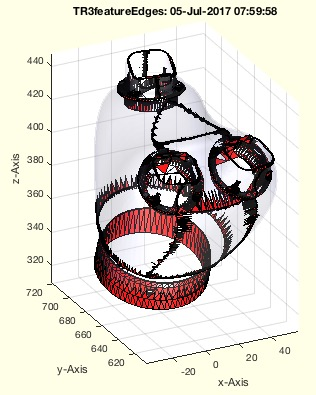% TR3featureEdges(TR3,alpha) - returns feature edge List plus edge attached facets% (by Tim Lueth, VLFL-Lib, 2017-JUL-05 as class: SURFACES)% % Auxiliary fnctn as starting point: % Simply calls featureEdges. It is not using edgeAttachments but an own % solution% (Status of: 2017-07-05)% % Introduced first in SolidGeometry 4.0% % [EL,Fi]=TR3featureEdges(TR3,alpha)% === INPUT PARAMETERS ===% TR3: Triangulation% alpha: feature edge angle% === OUTPUT RESULTS ======% EL: Feature Edge List% Fi: Index of TR3.ConnectivityList of attached facets% % EXAMPLE: % TR3featureEdges(SGsample(25),1)% % % Copyright 2017 Tim C. Lueth
###### TR3neighborsAngle(TR3)- returns the angle difference between a facet an its neigbors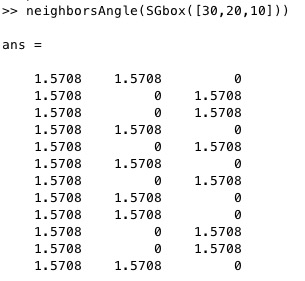% TR3neighborsAngle(TR3) - returns the angle difference between a facet an its neigbors% (by Tim Lueth, Video-Lib, 2017-JUL-04 as class: SURFACES)% % Calculated the difference angle between a surface and its neighbor % surfaces.% Typically used in combination with/to create the input for % fnctn neighborsAngleSurface (Status of: 2017-07-05)% % Introduced first in SolidGeometry 4.0% % See also: neighborsAngleSurface, diffangle% % [NAL,NBL,FNL]=TR3neighborsAngle(TR3)% === INPUT PARAMETERS ===% TR3: Triangulation or SG% === OUTPUT RESULTS ======% NAL: Facet Normal Angle List wrt to NBL% NBL: Neighbor List = neighbors(TR3)% FNL: Facet normal List = faceNormal(TR3)% % See also: neighborsAngleSurface, diffangle% % % Copyright 2017 Tim C. Lueth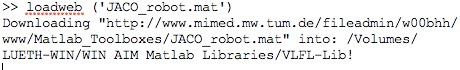% loadweb (mname,overwr,urlp) - loads mat files from an web url% (by Tim Lueth, VLFL-Lib, 2017-JUL-03 as class: AUXILIARY PROCEDURES)% % This fnctn reduces the size of the SG-Lib Toolbox since the '.mat' % files and the directories can be downloaded once into the user % directory.% if parameter overwr=false; the filename will be "downloaded_mname"% if parameter overwr=true; the filename will be "mname"% Try: % 'AAruffo_surf.mat' (3.6 MByte) = Geometry of some human arms% 'JACO_robot.mat' (6.1 MByte) = Geometry of the JACO robot structure% 'AIM_SGrobot.mat' (0.1 MByte) % 'AIM_Patientmodel.mat' ( 5MByte) = 216 x 216 x 26 Slices of a Human % Head CT Voxel model% 'ModulKegelradwelle_1.mat' (0.2 MByte)% 'NDI_STLs.mat' (0.1 MByte) = Geometry of the NDI mounting post for % spheres (Status of: 2017-07-26)% % Introduced first in SolidGeometry 4.0% % See also: load, webread% % loadweb(mname,[overwr,urlp])% === INPUT PARAMETERS ===% mname: name and extension of the file% overwr: true=overwrite; default is false.% urlp: web path; default is % 'https://www.mec.ed.tum.de/fileadmin/w00cbp/mimed/Matlab_Toolboxes/'% % EXAMPLE: % loadweb('JACO_robot.mat') % loads the downloaded file% loadweb('JACO_robot.mat',true) % replaces the original file by the % downloaded file% % See also: load, webread% % % Copyright 2017-2018 Tim C. Lueth